#### Concept of Steady-State Response of Electrodes

Introduction:

In measuring electrical bio-signals, the electrodes act as interface among the surface of skin and the input of recording amplifier. They essentially execute the task of transforming the ionic current related with electrical activity in the body into electronic current that is fed into the input of a recording amplifier. This is facilitated via the medium of an electrolyte in the form of coupling gel. As the signal of interest is electrical in nature and the amplifier and its related circuitry is electrical, it is necessary to know how the electrode will persuade the electrical behaviour and performance of measurement set-up. This signifies that it is significant to establish an electrical equivalent model for the electrode and hence the electrical behaviour of the measurement set-up can be examined. Only in this manner can the performance needs of the recording amplifier be established to make sure that the signal presented at the output of amplifier is an accurate and reliable representation of the electrical activity in the body that it is intended to observe.

The usual ECG electrodes shown below are pre-jelled with an electrolyte and hence this electrolyte makes contact with the skin whenever the electrode is positioned and adheres to it.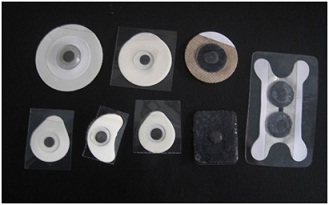Figure: Adhesive, Pre-jelled ECG Electrodes

Electrode Electrical Model:

The simplified model of electrode skin interface is as shown in figure below. When the electrolyte gel comes into contact with skin, the ionic chemical interaction establishes a polarisation potential that is symbolized by the dc voltage source, VPOL, as shown. The resistor RS symbolizes the series contact and lead resistance that is generally very small as compared to the impedances of other components. The capacitor CP symbolizes the capacitance formed by the contact region of the electrode on one side and the skin on other. The resistor RP symbolizes the conductance of coupling gel as a poor dielectric however as well the electrical interaction with the surface of skin. Such latter two elements thus appear in parallel.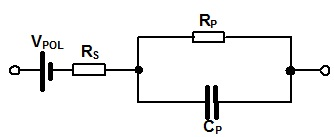Figure: A Simple Electrode Model

Frequency Response:

This is imperative to investigate the consequence of the equivalent circuit impedances on the frequency response of the total recording system. The front-end of this system can be treated as signal source feeding two electrodes, one on either leg of inputs to a differential amplifier as shown in figure below.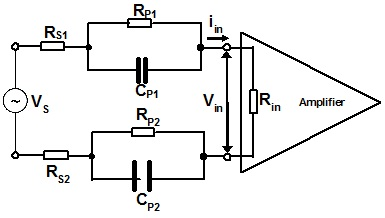Figure: Model of the Front End of the Recoding System

For present, the input impedance of the amplifier is taken as purely resistive. The polarisation potential is dc and therefore can be ignored from the view point of the ac analysis.

For steady state conditions assume s = jω then the transfer function is as follows:

Vin = iin Rin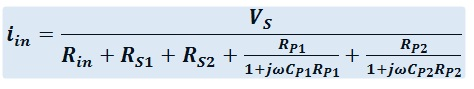Then the transfer function is written as: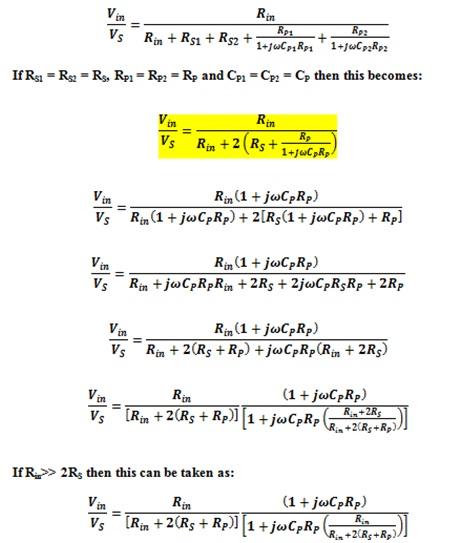The term on left hand side can be taken as the dc attenuation factor:

α = Rin/[Rin + 2(RS + RP)]

This signifies that the frequency response is of the form:

Vin/VS = α [{1+j(ω/ωZ)}/{ 1+j(ω/ωP)}]

Where: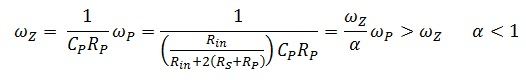By expressing the response in form of phase and magnitude gives: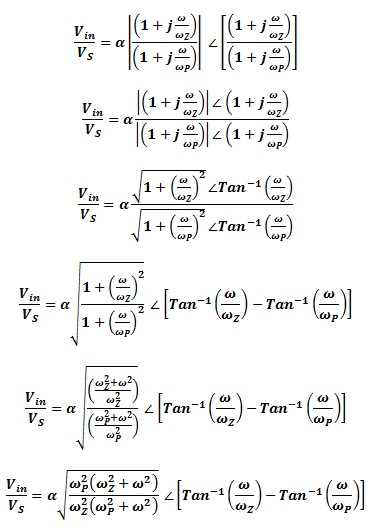It is a universal form of transfer function for such a pole-zero combination.

In this case with ωP = ωZ/α we have,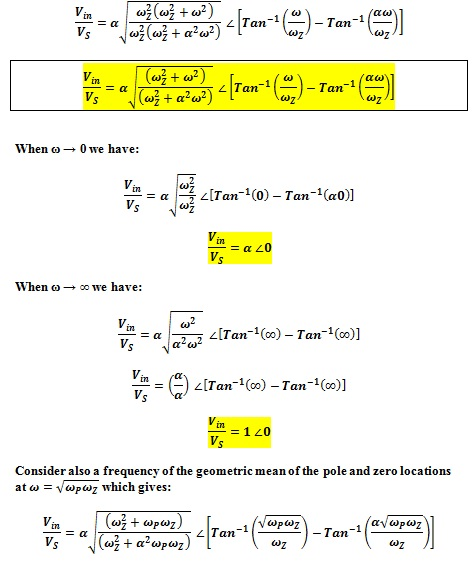However again with ωP = ωZ/α, this gives: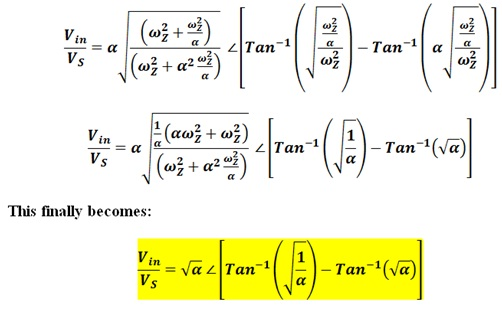The asymptotic values and the value at geometric mean of the response permit a Bode sketch of gain and phase to be plotted.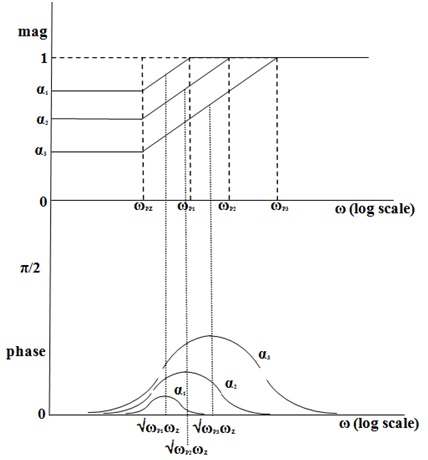Figure: Bode Diagram of the Frequency response of Electrode Model

Amplifier Input Impedance Requirements:

This can be seen that the high frequency magnitude is unity whereas the low frequency asymptote depends on the attenuation factor, α. Phase response is asymtotic to zero at low and high frequencies, however has a peak phase that as well depends on the attenuation factor α that is given as:

α = Rin/[Rin + 2(RS + RP)]

Note as well that the position of the zero frequency based only on the properties of electrode, whereas that of the pole based in addition on the attenuation factor α as: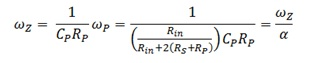The attenuation factor itself based on the input resistance of the amplifier, Rin.

General values for electrode components for the modern disposable adhesive electrodes are RS = 50Ω, RP = 200kΩ and CP = 0.5µF. Such values give the frequency of zero as:

fZ = 1/(2 Π CP RP) = 1/(2 x 3.14 x 0.5 x 10-6 x 2 x 105) = 10/6.28 = 15.9 Hz

It must be noted that this frequency is well in the spectrum of the ECG signal at low heart rates. The basic component in the spectrum of ECG at a heart rate of 40 bpm is 0.67 Hz that is employed in some regulation standards as the lower end of spectrum. The frequency of zero given above is more than twice this.

The frequency of pole depends on the degree of attenuation present, that is, on the value of α. For a range of values of α frequency of the pole can be computed as in Table I shown below. Value of the maximum phase in reality takes place at the frequency of geometric mean of the pole and zero and also based on the value of α and is shown in Table as well.

Table : Pole Frequency and Phase for Different Values of α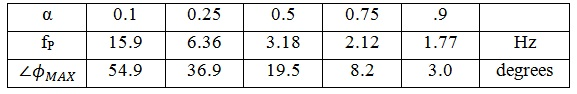Older performance specifications for electrocardiographs issued by bodies like American Heart Association (AHA) propose that the magnitude response in the bandwidth of the ECG must be flat to in a variation of ±0.5dB that corresponds to ±6% and must not have a phase shift more than that introduced by the single-pole, high-pass filter with a pole frequency of 0.05Hz. This filter has a phase shift of 5.6O at a frequency of 0.5Hz. In order to keep the attenuation less than 6 percent, the value of α requires to be higher than 0.94. This provides:

α ≥ = Rin/[ Rin + 2(RS + RP)] > 0.94

That means,

Rin > 0.94 Rin + 1.88 (RS + RP)

Or, 0.06 Rin > 1.88 (RS + RP)

And hence,

Rin > 31.3 (RS + RP)

For the values of such components given above as RS = 50Ω, RP = 200kΩ this signifies that:

Rin > 6.26 MΩ

This is simply attained with an amplifier input resistance of 10MΩ. This can be seen from Table above that an attenuation factor of 0.96 is related with a maximum phase shift of less than 3O which will thus as well satisfy the phase need of the performance standards. Though, it should be noted that electrodes containing values of the model components which might give much higher impedances than those above will need an amplifier containing a much higher input resistance than 10MΩ. Dry electrodes that do not need a coupling gel and which are the subject of current research fall into this group/category.

Obviously the universal solution is to form the amplifier input impedance as high as possible sand hence,

α → 1, ωP → ωZ and Vin/VS → 1∠0

Latest technology based Electrical Engineering Online Tutoring Assistance

Tutors, at the www.tutorsglobe.com, take pledge to provide full satisfaction and assurance in Electrical Engineering help via online tutoring. Students are getting 100% satisfaction by online tutors across the globe. Here you can get homework help for Electrical Engineering, project ideas and tutorials. We provide email based Electrical Engineering help. You can join us to ask queries 24x7 with live, experienced and qualified online tutors specialized in Electrical Engineering. Through Online Tutoring, you would be able to complete your homework or assignments at your home. Tutors at the TutorsGlobe are committed to provide the best quality online tutoring assistance for Electrical Engineering Homework help and assignment help services. They use their experience, as they have solved thousands of the Electrical Engineering assignments, which may help you to solve your complex issues of Electrical Engineering. TutorsGlobe assure for the best quality compliance to your homework. Compromise with quality is not in our dictionary. If we feel that we are not able to provide the homework help as per the deadline or given instruction by the student, we refund the money of the student without any delay.